0
862

# Direction Sense Test Questions For CMAT & TISSNET PDF

Download CMAT and TISSNET Direction Sense Test Questions and Answers PDF. Most expected questions for CMAT & TISSNET 2021 exams.

Question 1: Madhuri’s mother Meena had asked her to go to a vegetable shop and get some vegetables. To reach the vegetable shop, Meena has told the directions as follows.Take a right turn from our house and walk 20 metres, you will reach a junction, From there take a left turn and walk 10 metres, You will reach a Library. Take a right turn and walk 40 metres to reach the vegetable shop and also Madhuri’s house is facing to the North. What is the distance travelled by madhuri from her house to vegetable shop?

a) 75 meters

b) 90 meters

c) 60 meters

d) 70 meters

Question 2: Anish, Battu, Chandu, Dev, Eesha,  are standing in a row facing to the north. Some more information is given below about their positions.(i) Not more than 2 people are there between Anish ans Eesha.
(ii) The distance between Anish and Eesha is 30 meters.
(iii) Eesha is to the east of Anish.
(iv) Exactly 2 persons are there between Battu and Dev.
(v) The distance between Anish and Battu is 10meters.(vi) Dev is to the east of Eesha.(vii) Distance between any two persons is exactly 10 meters.What is the distance between the person sitting to the immediate right of Battu and Eesha?

a) 20 meters

b) 40 meters

c) 10 meters

d) 30 meters

Question 3: Raja has started to his friend’s home which is facing south at 7:00 am from his house in the  direction of his shadow and walks 20 meters and reaches a point x. From there he turns left and walk 15 meters straight and reaches the point y. From this point, he takes right turn and walks 20 more meters to reach his friends house.
What is the shortest distance between x and z?

a) 29 meters

b) 30 meters

c) 35 meters

d) 25 meters

Question 4: Raja has started to his friend’s home which is facing south at 7:00 am
from his house in the direction of his shadow and walks 20
meters and reaches a point x. From there he turns left and walk 15
meters straight and reaches the point y. From this point, he takes right
turn and walks 20 more meters to reach his friends house.
If he takes the shortest distance from X to friends home, then what is the total distance travelled by Raja?

a) 35 meters

b) 55 meters

c) 45 meters

d) 65 meters

Question 5: Rahim is standing outside his school at point R facing towards North. He started walking 10 meters and took a left turn and walked 5 meters. From there he took right turn and walked 8 meters to reach point “B”. From B , he walked 15 meters towards his right and reaches point “C” then he took a right turn again and walked 18 meters to reach Ice cream shop at “K”. Now, what is the distance between his school and ice cream shop?

a) 5 meters

b) 10meters

c) 20meters

d) 7 meters

e) e

Question 6: Rahim is standing outside his school at point R facing towards North. He
started walking 10 meters and took a left turn and walked 5 meters.
From there he took right turn and walked 8 meters to reach point “B”.
From B , he walked 15 meters towards his right and reaches point “C”
then he took a right turn again and walked 18 meters to reach Ice cream
shop at “K”. Now, what is the distance between his school and ice cream
shop?
What is the nearest value of the shortest between B and K

a) 23

b) 25

c) 26

d) 27

Question 7: East is coded as “$\ \ \ +\$”
West is coded as “$\ -\$”
North is coded as “$\ \ \triangle\$”
South is coded as “$\ \ \%\$”
A is 5m “$\ +\$” to B
C is 10m “$\ \ \triangle\$”to B
F is  5m “$\ \ \%\$” of A
D is 15m “$\ -\$” of C
The shortest distance between F and C is ?

a) $\ 10\sqrt{\ 2}\ +\ 10$

b) $5\sqrt{\ 2}\ +\ 10$

c) $7\sqrt{\ 2}\ +\ 15$

d) $5\sqrt{\ 2}\ +\ 13$

Question 8: East is coded as “$\ +\$”
West is coded as “$\ -\$”
North is coded as “$\ \frac{\ }{ }\triangle\$”
South is coded as “$\ \ \%\$”
A is 5m “$\ +\$” to B
C is 10m  “$\ \frac{\ }{ }\triangle\$”to B
F is 5m “$\ \ \%\$” of A
D is 15m “$\ -\$” of C
D is in what direction wrt B?

a) North West

b) North East

c) South-West

d) East

Question 9: Seeta is facing towards North. She was asked to turn her right and walk 5m to meet vamshi. From there she was asked to turn left and walk 7m to meet raghu.From there she was asked to turn left and travel 10m to meet Lakshmi. From there she was asked to turn left and walk 19m to meet Manisha. Now she has to go back to her starting point from Manisha’s place. For that what would be the shortest distance she has to travel?

a) 14m

b) 13m

c) 10m

d) 5m

Question 10: Seeta is facing towards North. She was asked to turn her right and walk
5m to meet vamshi. From there she was asked to turn left and walk 7m to
meet raghu.From there she was asked to turn left and travel 10m to meet
Lakshmi. From there she was asked to turn left and walk 19m to meet
Manisha.  In which direction is Latha’s  with respect to Seeta’s starting point?

a) East

b) North-West

c) South-East

d) West

e) e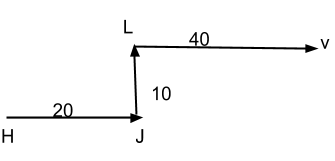From the above question, we can draw this route map from madhuri’s house to vegetable shop.

The total distance travelled by Madhuri is 20+10+40=70meters.

From (i), (iii), and (iv)  we can conclude that

we can also conclude from (v),(vi) that

Dev can be immediate east of Eesha but from (iv) it is given that there are exactly two persons between Battu and Dev

So,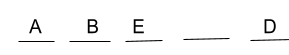but from (vii) we know that the exact distance between two persons is 10 metres and from (ii) it is given that the distance between Anish and Eesha is 30 meters. So, Eesha is third to the right of Anish

so the formation will be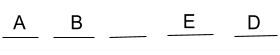Now the remaining person is Chandu. She will be standing to the east of Battu.

Therefore, the final formation is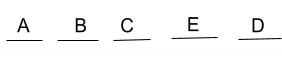The person sitting immediate to the east of Battu is chandu

given that the distance between any two persons is 10 meters  so, distance between Chandu and Eesha is 10 meters.

We know that,

if a man faces south, his shadow will be on his right during sunrise and on his left during sunset.

so, here the house is facing south so the shadow is on his right

Given that he walks to the same side of his shadow. That means he is walking towards his right.

first he walked 20 meters and then he took a left turn and walked 15 meters to reach y.

From here he took a right turn and walked 20 meters to reach his friends home.

So, the final direction diagram can be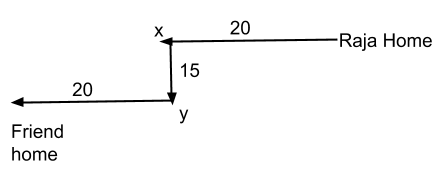The shortest distance between X and friends home is

$\sqrt{\ 15^2+20^2}$= 25

We know that,

if a man faces south, his shadow will be on his right during sunrise and on his left during sunset.

so, here the house is facing south so the shadow is on his right.

Given that he walks to the same side of his shadow. That means he is walking towards his right.

first he walked 20 meters and then he took a left turn and walked 15 meters to reach y.

From here he took a right turn and walked 20 meters to reach his friends home.

So, the final direction diagram can beThe shortest distance between X and friends home is

$\sqrt{\ 15^2+20^2}$= 25

so the total distance is the sum of Raja home to x and X to friends home

i.e,=> 20+25=45meters.

From the given directions, we can say conclude the direction map diagram as follows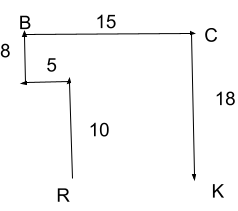Now, given that the distance between C and K is 18

and we can extend the line from R which meets the line BC at P so the distance between PR is 18.

so, the distance between PC is same as the distance between RK i.e; 10 meters.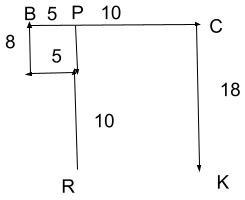From the given directions, we can say conclude the direction map diagram as followsNow, given that the distance between C and K is 18

and we can extend the line from R which meets the line BC at P so the distance between PR is 18.

so, the distance between PC is same as the distance between RK i.e; 10 meters.The shortest distance between B and K is $\sqrt{\ 15^2+18^2}$=$\sqrt{\ 549}$

the nearest whole number to $\sqrt{\ 549}$ is 23

therefore answer is 23 option a

A is 5m “$\ \ \ +\$” to B means A is 5m east of B

C is 10m “$\ \ \triangle\$” to B means C is 10m North of B

F is 5m “$\ \ \%\$” of A means F is 5m South of A

D is 15m “$\ -\$” of C means D is 15m west of C

following these instructions we can draw the direction map as follows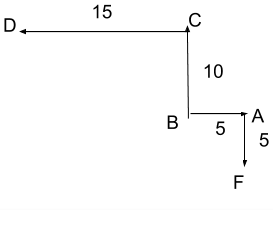The shortest distance between F and C is BF+BC

BF= $\sqrt{\ 5^2\ +\ 5^2}$=$5\sqrt{\ 2}$

BC= 10

FC=$5\sqrt{\ 2}\ +\ 10$

A is 5m “$\ +\$” to B means A is 5m east of B

C is 10m “$\ \frac{\ }{ }\triangle\$” to B means C is 10m North of B

F is 5m “$\ \ \%\$” of A means F is 5m South of A

D is 15m “$\ -\$” of C means D is 15m west of C

following these instructions we can draw the direction map as followsD is to North west of B

From the above given directions, we can draw the direction map as follows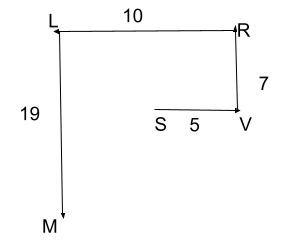The shortest distance between M and S is $\sqrt{\ 12^2\ +\ 5^2}$ =13